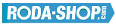# Remote ControlManufacturer Product Image Item Name Price### 627

Miniature Metric Series Chrome Steel Open(No Shields) Dimension d=7.00 Dimension D=22.00 Dimension B=7.00 Dinamic Load Rating=3287 Static Load Rating=1379 Weight=0.014gr. Speed limit Grease=30.0 Speed limit Oil=36.0
inside diameter = 7.00
outside diameter = 22.00
width = 7.00
€2.24### 627-2RS

Miniature Metric Series Chrome Steel Rubber Seals on Both sides Dimension d=7.00 Dimension D=22.00 Dimension B=7.00 Dinamic Load Rating=3287 Static Load Rating=1379 Weight=0.014gr. Speed limit Grease=30.0 Speed limit Oil=36.0
inside diameter = 7.00
outside diameter = 22.00
width = 7.00
€2.24### 627-2Z

Miniature Metric Series Chrome Steel Metallic Shields on both sides Dimension d=7.00 Dimension D=22.00 Dimension B=7.00 Dinamic Load Rating=3287 Static Load Rating=1379 Weight=0.014gr. Speed limit Grease=30.0 Speed limit Oil=36.0
inside diameter = 7.00
outside diameter = 22.00
width = 7.00
€2.24### 628

Miniature Metric Series Chrome Steel Open(No Shields) Dimension d=8.00 Dimension D=24.00 Dimension B=8.00 Dinamic Load Rating=3333 Static Load Rating=1423 Weight=0.018gr. Speed limit Grease=28.0 Speed limit Oil=34.0
inside diameter = 8.00
outside diameter = 24.00
width = 8.00
€2.24### 628-2RS

Miniature Metric Series Chrome Steel Rubber Seals on Both sides Dimension d=8.00 Dimension D=24.00 Dimension B=8.00 Dinamic Load Rating=3333 Static Load Rating=1423 Weight=0.018gr. Speed limit Grease=28.0 Speed limit Oil=34.0
inside diameter = 8.00
outside diameter = 24.00
width = 8.00
€2.24### 628-2Z

Miniature Metric Series Chrome Steel Metallic Shields on both sides Dimension d=8.00 Dimension D=24.00 Dimension B=8.00 Dinamic Load Rating=3333 Static Load Rating=1423 Weight=0.018gr. Speed limit Grease=28.0 Speed limit Oil=34.0
inside diameter = 8.00
outside diameter = 24.00
width = 8.00
€2.24### 62800

Thin Section Bearings Chrome Steel Open(No Shields) Dimension d=10.00 Dimension D=19.00 Dimension B=6.00 Dinamic Load Rating=0 Static Load Rating=0 Weight=0.007gr. Speed limit Grease=0.0 Speed limit Oil=-
inside diameter = 10.00
outside diameter = 19.00
width = 6.00### 62800-2RS

Thin Section Bearings Chrome Steel Rubber Seals on Both sides Dimension d=10.00 Dimension D=19.00 Dimension B=6.00 Dinamic Load Rating=0 Static Load Rating=0 Weight=0.007gr. Speed limit Grease=0.0 Speed limit Oil=-
inside diameter = 10.00
outside diameter = 19.00
width = 6.00
€11.98### 62800-2Z

Thin Section Bearings Chrome Steel Metallic Shields on both sides Dimension d=10.00 Dimension D=19.00 Dimension B=6.00 Dinamic Load Rating=0 Static Load Rating=0 Weight=0.007gr. Speed limit Grease=0.0 Speed limit Oil=-
inside diameter = 10.00
outside diameter = 19.00
width = 6.00### 629

Miniature Metric Series Chrome Steel Open(No Shields) Dimension d=9.00 Dimension D=26.00 Dimension B=8.00 Dinamic Load Rating=4575 Static Load Rating=1983 Weight=0.022gr. Speed limit Grease=28.0 Speed limit Oil=34.0
inside diameter = 9.00
outside diameter = 26.00
width = 8.00
€2.24